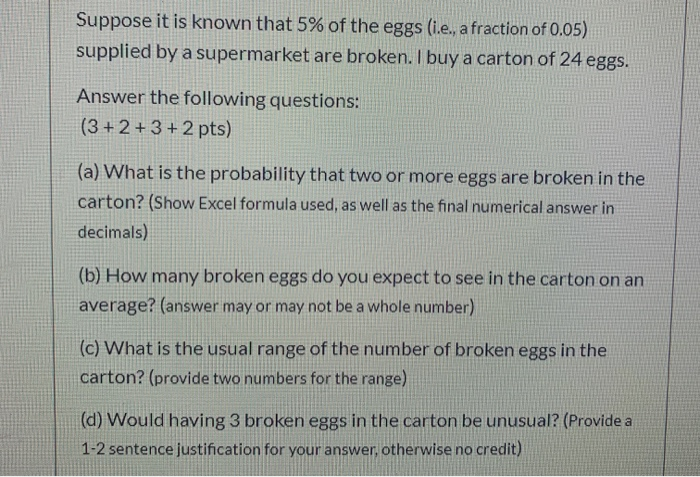# Question Suppose it is known that 5% of the eggs (i.e., a fraction of 0.05) supplied by a supermarket are broken. I buy a carton of 24 eggs. Answer the following questions: (3 + 2+ 3+ 2 pts) (a) What is the probability that two or more eggs are broken in the carton? (Show Excel formula used, as well as the final numerical answer in decimals) (b) How many broken eggs do you expect to see in the carton on an average? (answer may or may not be a whole number) (c) What is the usual range of the number of broken eggs in the carton? (provide two numbers for the range) (d) Would having 3 broken eggs in the carton be unusual? (Provide a 1-2 sentence justification for your answer, otherwise no credit)9CCXJI The Asker · Probability and StatisticsTranscribed Image Text: Suppose it is known that 5% of the eggs (i.e., a fraction of 0.05) supplied by a supermarket are broken. I buy a carton of 24 eggs. Answer the following questions: (3 + 2+ 3+ 2 pts) (a) What is the probability that two or more eggs are broken in the carton? (Show Excel formula used, as well as the final numerical answer in decimals) (b) How many broken eggs do you expect to see in the carton on an average? (answer may or may not be a whole number) (c) What is the usual range of the number of broken eggs in the carton? (provide two numbers for the range) (d) Would having 3 broken eggs in the carton be unusual? (Provide a 1-2 sentence justification for your answer, otherwise no credit)
More
Transcribed Image Text: Suppose it is known that 5% of the eggs (i.e., a fraction of 0.05) supplied by a supermarket are broken. I buy a carton of 24 eggs. Answer the following questions: (3 + 2+ 3+ 2 pts) (a) What is the probability that two or more eggs are broken in the carton? (Show Excel formula used, as well as the final numerical answer in decimals) (b) How many broken eggs do you expect to see in the carton on an average? (answer may or may not be a whole number) (c) What is the usual range of the number of broken eggs in the carton? (provide two numbers for the range) (d) Would having 3 broken eggs in the carton be unusual? (Provide a 1-2 sentence justification for your answer, otherwise no credit)